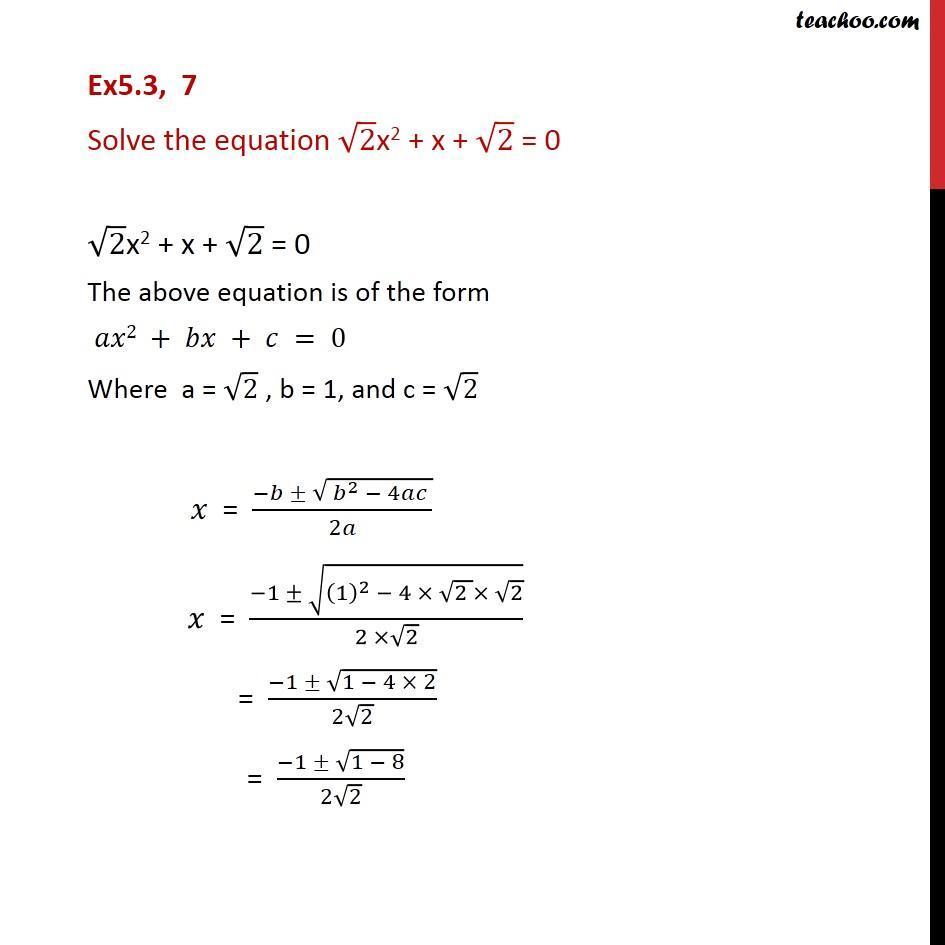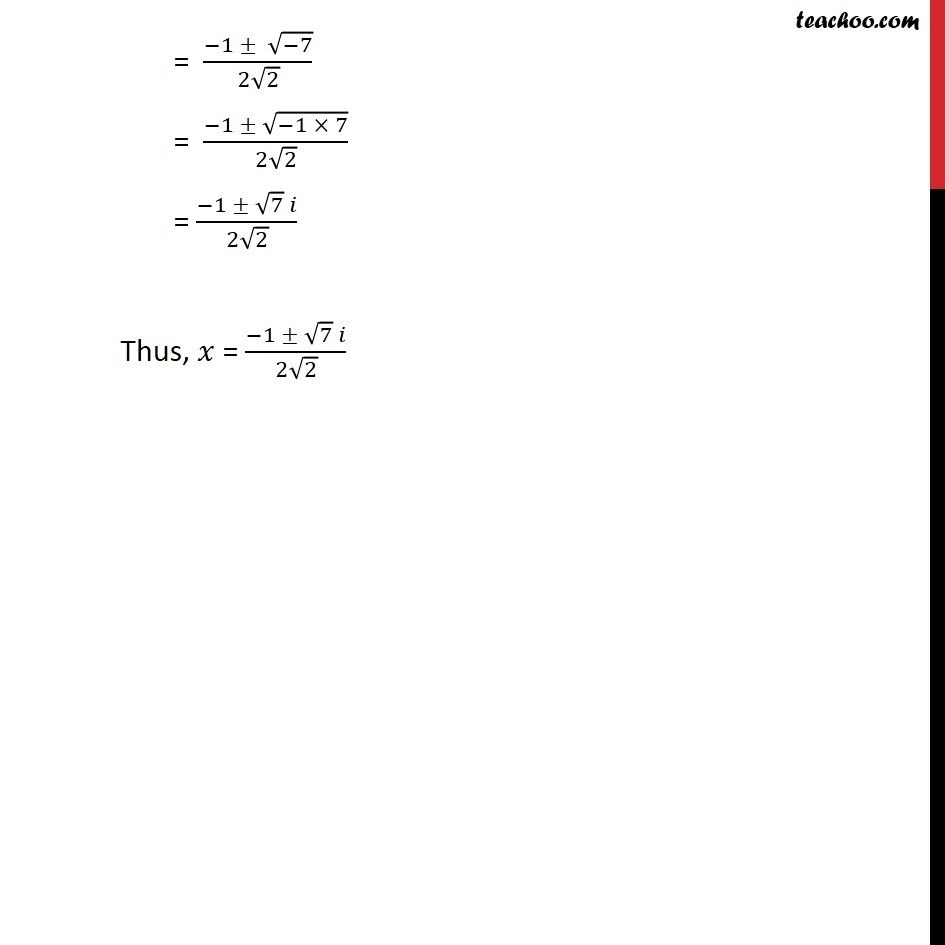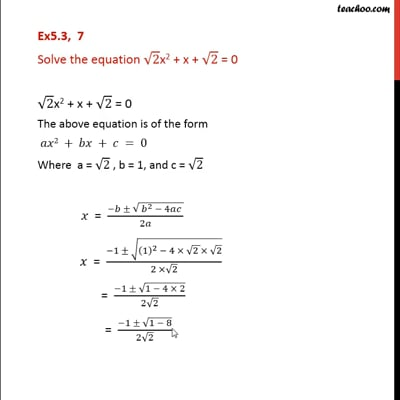Chapter 4 Class 11 Complex Numbers
Serial order wiseThis video is only available for Teachoo black users

Learn in your speed, with individual attention - Teachoo Maths 1-on-1 Class

### Transcript

Ex5.3, 7 Solve the equation 2x2 + x + 2 = 0 2x2 + x + 2 = 0 The above equation is of the form 2 + + = 0 Where a = 2 , b = 1, and c = 2 = ( ( ^2 4 ))/2 = ( 1 ((1)^2 4 (2 ) 2) )/(2 2) = ( 1 (1 4 2))/(2 2) = ( 1 (1 8))/(2 2) = ( 1 ( 7))/(2 2) = ( 1 ( 1 7))/(2 2) = ( 1 7 )/(2 2) Thus, = ( 1 7 )/(2 2)